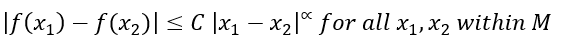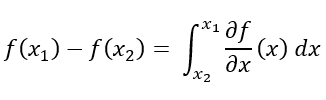# Lipschitz Function

## Lipschitz Function: Definition

Lipschitz Functions are important in mathematics because they guarantee the existence and uniqueness of solutions for differential equations. The proof in identifying a Lipschitz Function makes sure that there is not a location where that function is infinitely steep (non-differentiable).

For a function f(x) that has a domain within the closed interval M that is [x1, x2], consisting of only real numbers, the function f (x) is said to be a Lipschitz Function if it can satisfy the Lipschitz Condition (Walker, 2019).

The Lipschitz Condition on f exists if there are two positive constants C and α such that:Where C is the Lipschitz Constant. The function f (x) is then said to satisfy the Lipschitz Condition of order α. We can then state that f(x) can be represented as a Lipschitz Function of order α.

The inequality can be trivially satisfied if the Lipschitz Condition is tested using the same x value for x1, x2. If x1 ≠ x2, then the ratio of absolute differences between two y-values and two x-values should equate to a constant that is less than or equal to C.

## Proof for the Lipschitz Condition

Starting with the fundamental theorem of calculus, for all x1, x2 within the interval [x1, x2], the difference of two y-values for a function f can be represented as:Applying the absolute value property to both sides allows for the inequality to be present between the difference of y-values and the area underneath the curve for f(x):And since the partial derivative in the integral is bounded for all x1, x2 within the interval [x1, x2], the bounding of derivable points within f(x) allows for the partial derivative to be some positive constant C.Notice that the order α is set to equal 1 because the Lipschitz Condition is being done on the first function order of function f(x) that contains no repeating zeros. The order α gets accounted for when testing for the satisfaction of a function to the Hölder Condition for order α < 0 (Ricco, 2004).

## Properties of Lipschitz Functions

If a function satisfies the Lipschitz Condition, that function is uniformly continuous on the set interval that was tested. In simpler wording, the inequality above aims to have the absolute difference of two x values to be equal or greater than the absolute difference between the Lipschitz Function’s y values.

If the differences can be evaluated, then f(x) is continuous so that any evaluation of two chosen coordinates within f(x) is possible. Keeping the difference in x values greater than the y values for function f(x) removes the worry of an non-differentiable point.

Since a Lipschitz Function is uniformly continuous and differentiable, a Lipschitz Function mimics that of functions that pass the Mean Value Theorem.

## Usefulness in Mathematics

Within the realm of Differential Equations, there are equations that carry the property of being solved for a solution that is unique for a given initial value. To guarantee the existence and uniqueness of this solution, the differential equation must have the Lipschitz Condition be true to itself. If the function(s) within a differential equation fails to be Lipschitz Functions, then the existence (and thus uniqueness of such a solution) cannot be found due to non-differentiability.

The Lipschitz Condition can be visually understood by the Cone Condition that is made up of two lines whose angle satisfies the equation:And takes the depiction below (Hu, 2004). The lines L1 and L2 must exist for every point for the function f(x) to have continuity and differentiability existing within f(x). Each point that the lines pass through appears only once within the Cone Condition. Thus visualizes the uniqueness of that solution for f(x).For functions that fail to be Lipschitz Functions, understanding the Lipschitz Function’s Condition backward can help explain why. If a constant C does not exist for the inequality within Lipschitz Condition, then the following logical statements are true:

1. C tends to approach infinity.
2. For C to equal infinity, the value for θ and θ/2 equals 0.
3. With the angle formed by lines L1 and L2 equaling to 0, the cone disappears.
4. The disappearance of the cone indicates that although a solution can exist for f(x), the uniqueness of that solution is lost due to it being within both lines.

## References

Hu, Jishan, and Wei-Ping Li. Theory of Ordinary Differential Equations – Existence, Uniqueness and Stability. The Hong Kong University of Science and Technology, 2004.

Ricco, Lorianne. “Lipschitz Functions.” MATH3912 – Junior Seminar in Mathematics, Seton Hall University , 4 Feb. 2004, pirate.shu.edu/~wachsmut/Teaching/MATH3912/Projects/papers/ricco_lipschitz.pdf.

Walker, Homer. “Lipschitz Continuity.” Basic Real Analysis – MA 500, Worcester Polytechnic Institute, 26 Feb. 2019, users.wpi.edu/~walker/MA500/HANDOUTS/LipschitzContinuity.pdf.

CITE THIS AS:
Stephanie Glen. "Lipschitz Function" From StatisticsHowTo.com: Elementary Statistics for the rest of us! https://www.statisticshowto.com/lipschitz-function/